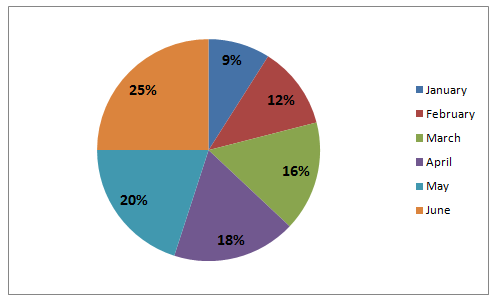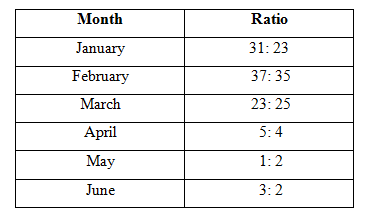# SBI Clerk Prelims 2021 Quantitative Aptitude Questions (Day-35)

Dear Aspirants, Our IBPS Guide team is providing new series of Quantitative Aptitude Questions for SBI Clerk Prelims 2020 so the aspirants can practice it on a daily basis. These questions are framed by our skilled experts after understanding your needs thoroughly. Aspirants can practice these new series questions daily to familiarize with the exact exam pattern and make your preparation effective.

Start Quiz

Directions (01-05): Study the following information carefully and answer the questions given below.

The below chart shows the percentage wise distribution of the number of laptops sold by a shopkeeper during six monthsTotal number of laptops sold = 1200

The below table shows the ratio between the number of laptops sold of company A and company B during six months respectively1) If 25% of the laptops sold by company A during March were sold at a discount, then how many laptops of company A during that month were sold without a discount?

A.24

B.36

C.57

D.69

E.None of these

2) If the shopkeeper earned a profit of Rs.800 on each laptop of company B during January, what was his total profit earned on selling the laptops of that company during the same month?

A.Rs.24600

B.Rs.36800

C.Rs.45800

D.Rs.87900

E.None of these

3) The number of laptops sold by company A during February is approximately what percent of the number of laptops sold by company A during May?

A.95%

B.97%

C.93%

D.94%

E.98%

4) What is the ratio of number of laptops sold by company B during June to those sold during April by the same company?

A.4: 5

B.5: 4

C.1: 3

D.3: 1

E.None of these

5) What is the total number of laptops sold by company A during February and April together?

A.160

B.178

C.180

D.194

E.None of these

Simplification

Directions (06-10):  What value should come in the place of (?) in the following questions.

6) 70 % of 540 – 120 % of 350 = ? * 6

A.-6

B.-5

C.-7

D.-8

E.–11

7) 513 ÷ 27+ ? = 55% of 780

A.380

B.400

C.370

D.410

E.390

8) (1170 ÷ 6) – 12 * 6 + 9 * √9= ?

A.120

B.140

C.180

D.110

E.150

9) 16 * ? = √2304 * (208 ÷ 13)

A.45

B.48

C.51

D.42

E.55

10) √3969 – √2601 + √784 = ?

A.50

B.60

C.30

D.40

E.20

No. of laptops sold in March = 16% of 1200 = 192

No. of laptops sold by A in March = 23/48 * 192 = 92

Required number of laptops = 75/100 * 92 = 69

No. of laptops sold in Jan = 9% of 1200 = 108

No. of laptops sold by B in Jan = 23/54 * 108 = 46

Total profit = 46 * 800 = Rs.36800

Required % = (12/100 * 37/72 * 1200)/(20/100 * 1/3 * 1200) * 100

= 74/80 * 100

= 92.5%

Required ratio = (25/100 * 2/5 * 1200): (18/100 * 4/9 * 1200)

= 5: 4

Required total = 12/100 * 37/72 * 1200 + 18/100 * 5/9 * 1200

= 74 + 120 = 194

70 % of 540 – 120 % of 350 = ? * 6

378 – 420 = ? * 6

? = -7

513 ÷ 27 + ? = 55% of 780

19 + ? = 429

? = 410

(1170 ÷ 6) – 12 * 6 + 9 * √9 = ?

195 – 72 + 27 = 0

? = 150

16 * ? = √2304 * (208 ÷ 13)

16 * ? = 48 * 16

? = 48

√3969 – √2601 + √784 = ?

63 – 51 + 28 = ?

? = 40

 Check Here to View SBI Clerk Prelims 2021 Quantitative Aptitude Questions Day – 34 Day – 33 Day – 32
5 1 vote
Rating